# Selina Solutions Concise Maths Class 7 Chapter 1: Integers Exercise 1B

Selina Solutions Concise Maths Class 7 Chapter 1 Integers Exercise 1B explains the concept of division of integers and properties of division of integers. Students can improve their knowledge about the concepts by regularly practising the problems. It will help students answer the tricky questions that would appear in the annual exam. Students who find it difficult in solving the exercise wise problems can download the PDF of solutions curated by the faculty at BYJU’S. For a better idea of the concepts, Selina Solutions Concise Maths Class 7 Chapter 1 Integers Exercise 1B PDF links are given below, for free download.

## Selina Solutions Concise Maths Class 7 Chapter 1: Integers Exercise 1B Download PDF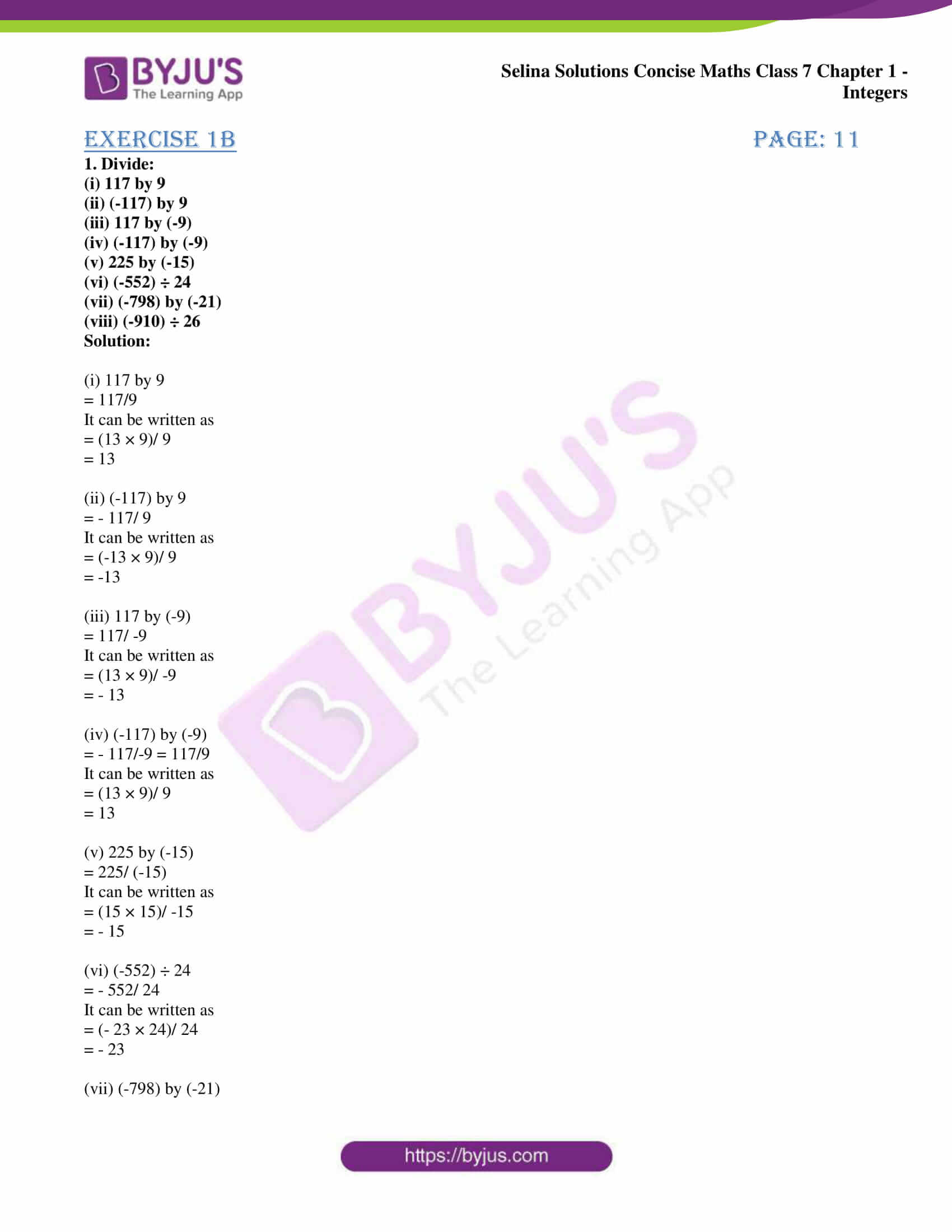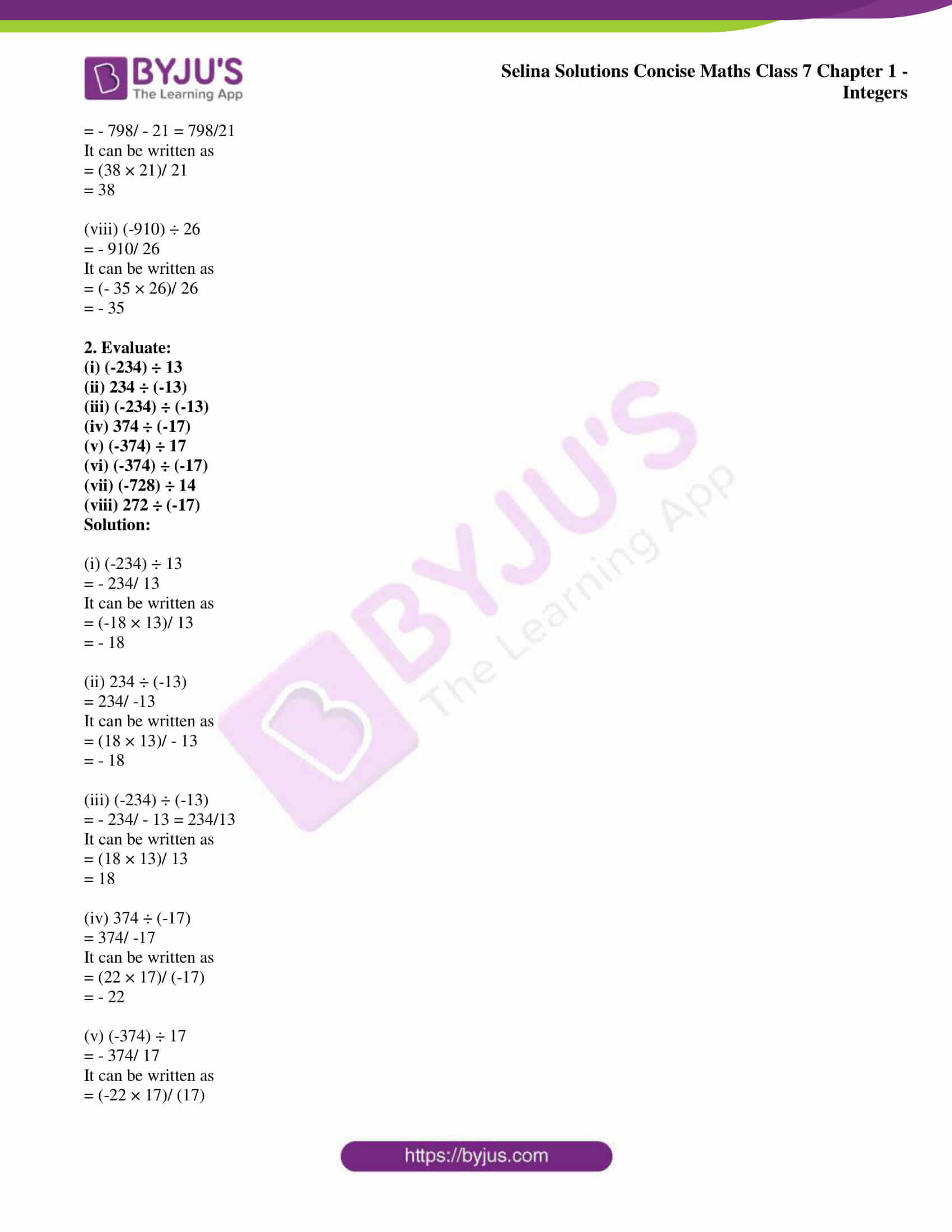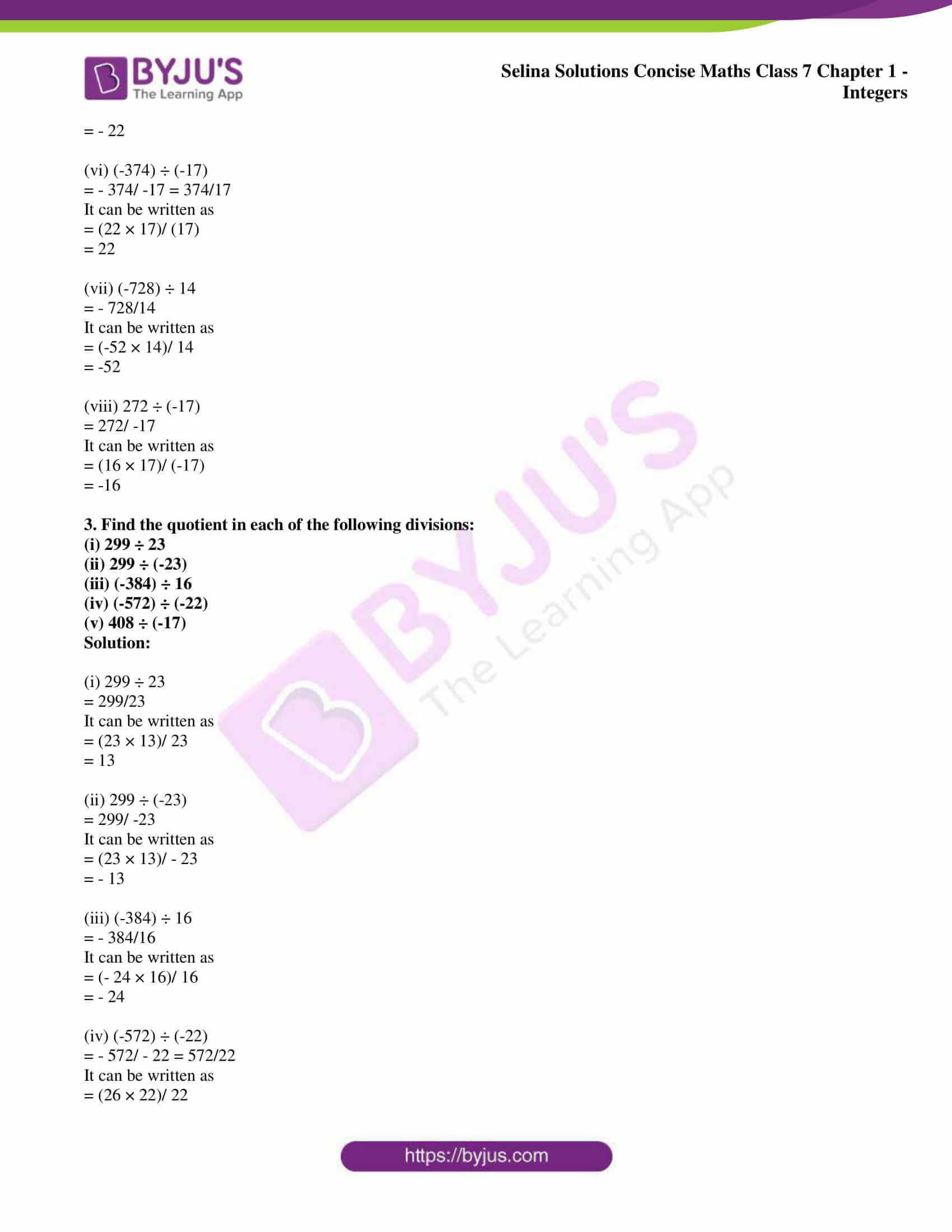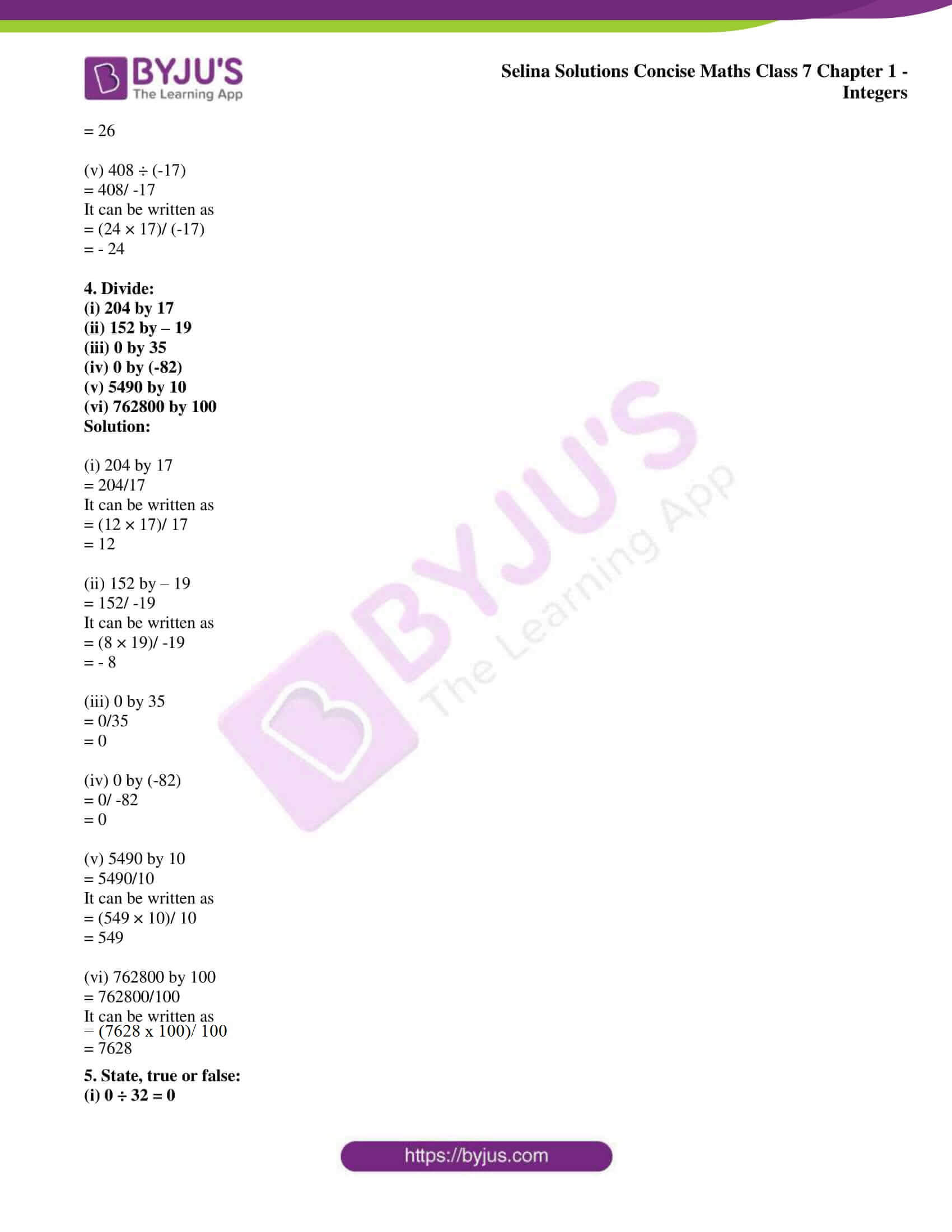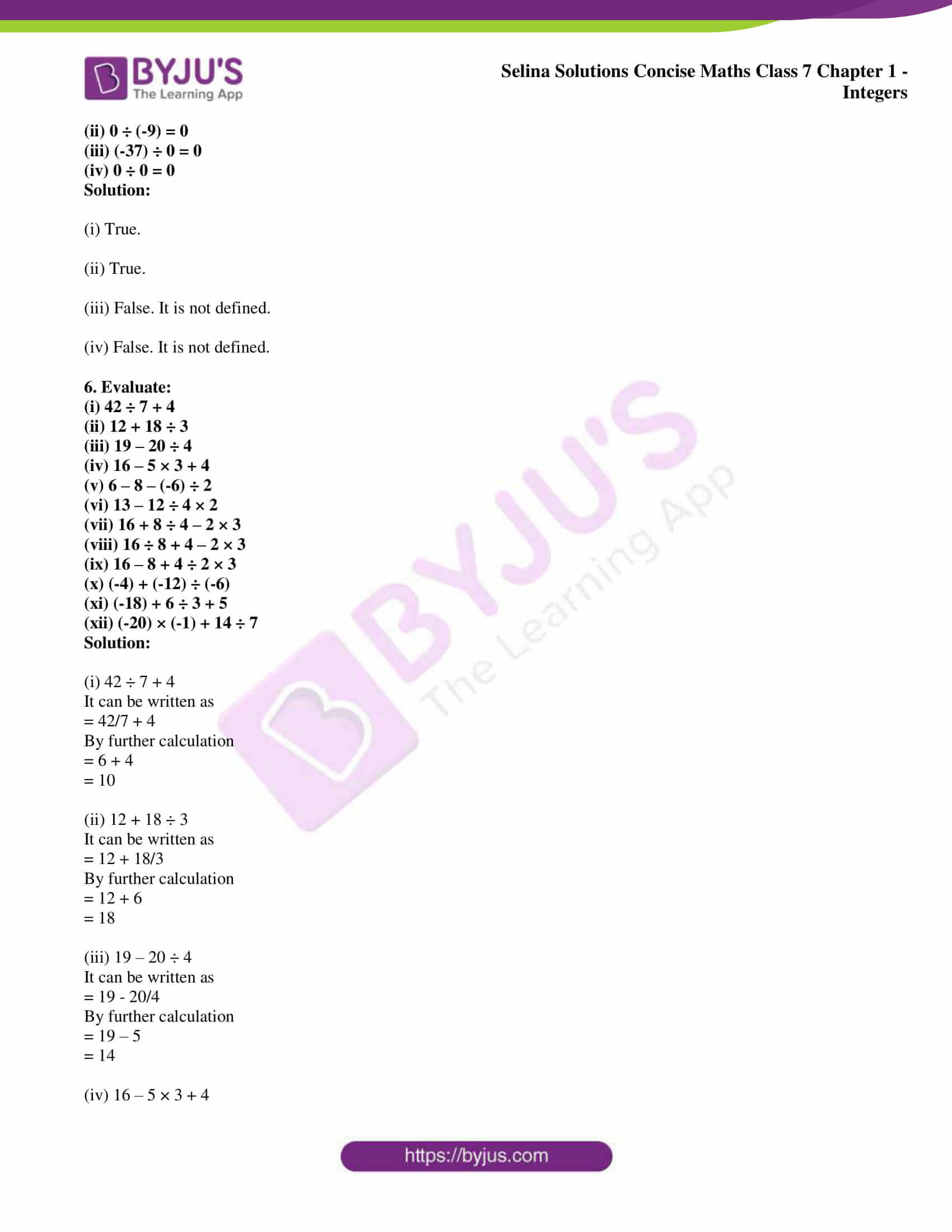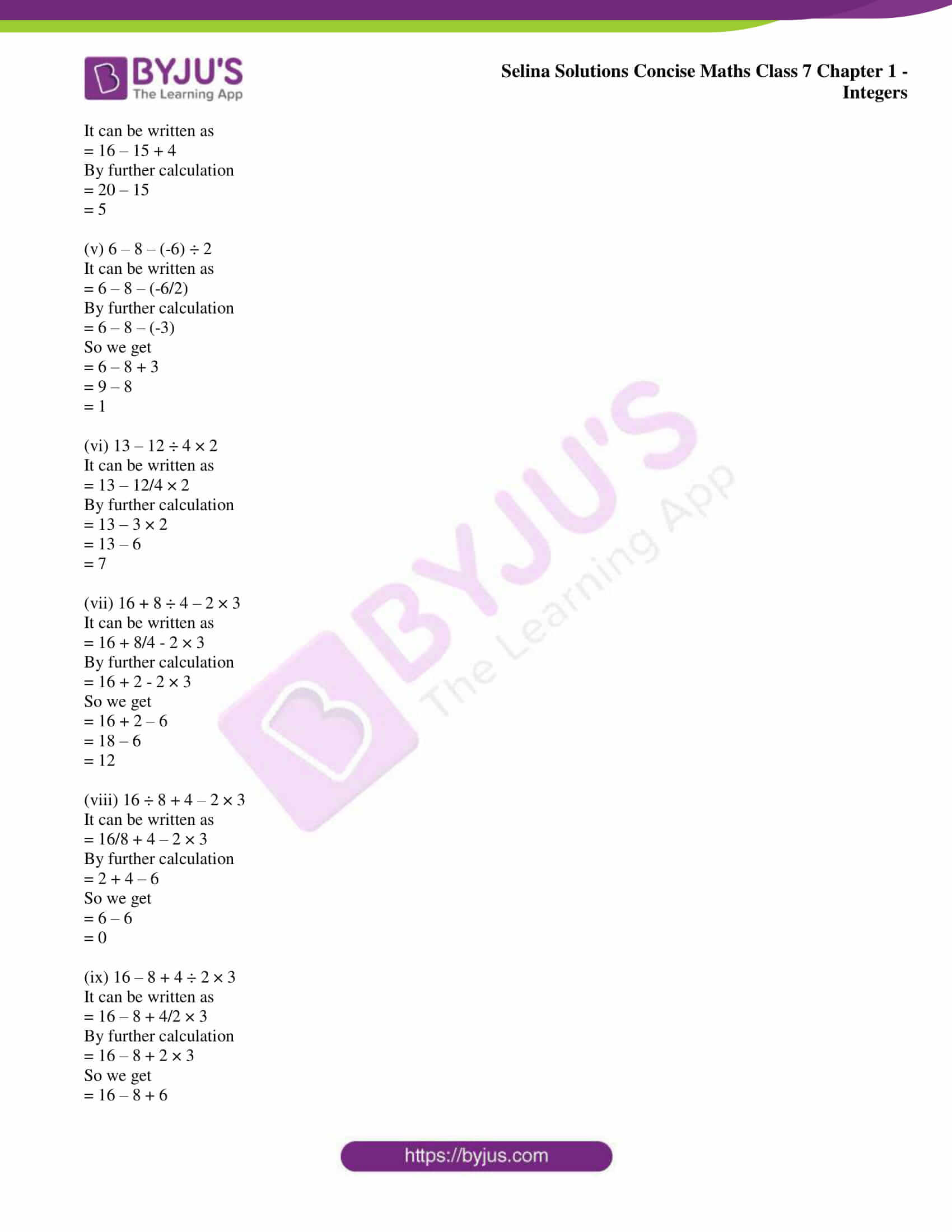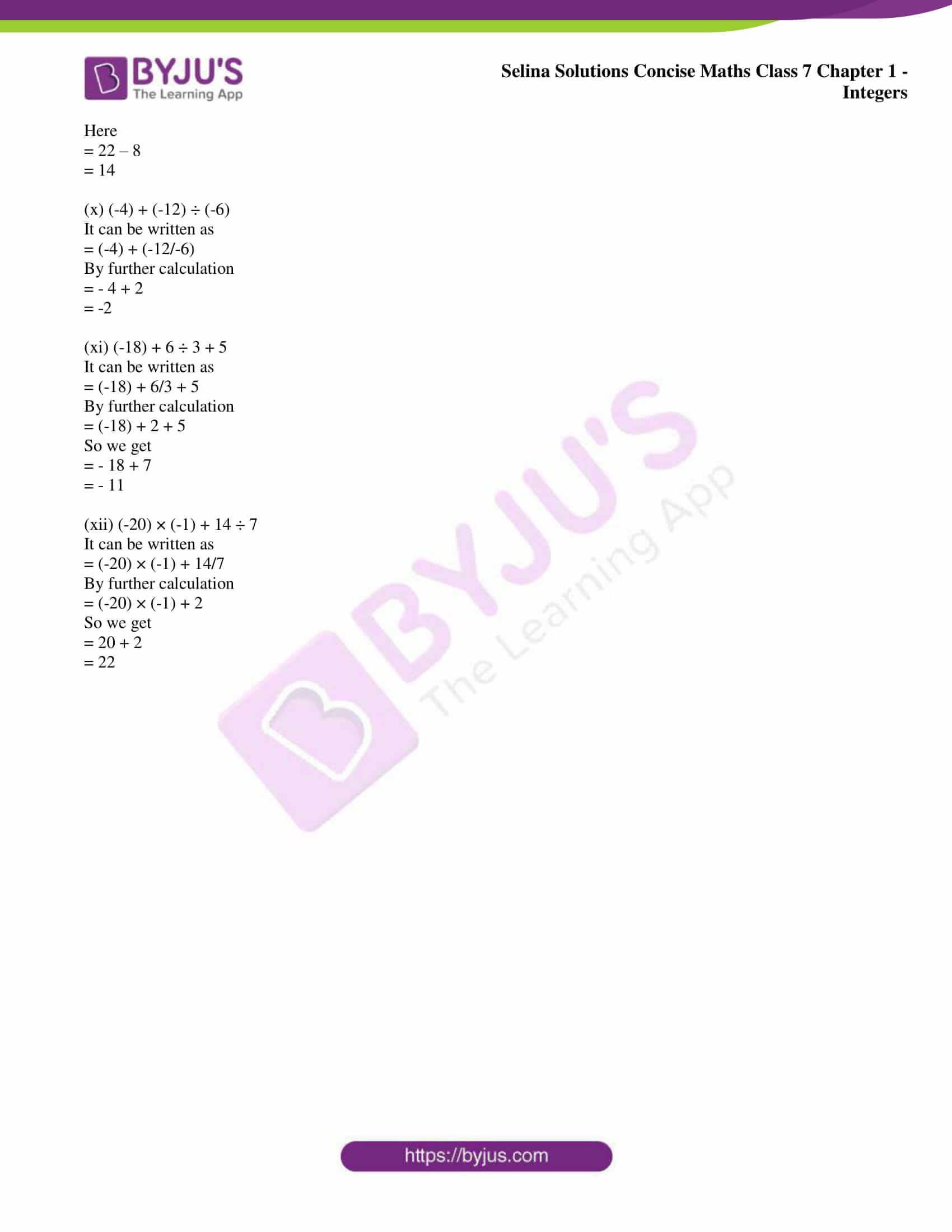### Access other exercises of Selina Solutions Concise Maths Class 7 Chapter 1: Integers

Exercise 1A Solutions

Exercise 1C Solutions

Exercise 1D Solutions

### Access Selina Solutions Concise Maths Class 7 Chapter 1: Integers Exercise 1B

1. Divide:

(i) 117 by 9

(ii) (-117) by 9

(iii) 117 by (-9)

(iv) (-117) by (-9)

(v) 225 by (-15)

(vi) (-552) ÷ 24

(vii) (-798) by (-21)

(viii) (-910) ÷ 26

Solution:

(i) 117 by 9

= 117/9

It can be written as

= (13 × 9)/ 9

= 13

(ii) (-117) by 9

= – 117/ 9

It can be written as

= (-13 × 9)/ 9

= -13

(iii) 117 by (-9)

= 117/ -9

It can be written as

= (13 × 9)/ -9

= – 13

(iv) (-117) by (-9)

= – 117/-9 = 117/9

It can be written as

= (13 × 9)/ 9

= 13

(v) 225 by (-15)

= 225/ (-15)

It can be written as

= (15 × 15)/ -15

= – 15

(vi) (-552) ÷ 24

= – 552/ 24

It can be written as

= (- 23 × 24)/ 24

= – 23

(vii) (-798) by (-21)

= – 798/ – 21 = 798/21

It can be written as

= (38 × 21)/ 21

= 38

(viii) (-910) ÷ 26

= – 910/ 26

It can be written as

= (- 35 × 26)/ 26

= – 35

2. Evaluate:

(i) (-234) ÷ 13

(ii) 234 ÷ (-13)

(iii) (-234) ÷ (-13)

(iv) 374 ÷ (-17)

(v) (-374) ÷ 17

(vi) (-374) ÷ (-17)

(vii) (-728) ÷ 14

(viii) 272 ÷ (-17)

Solution:

(i) (-234) ÷ 13

= – 234/ 13

It can be written as

= (-18 × 13)/ 13

= – 18

(ii) 234 ÷ (-13)

= 234/ -13

It can be written as

= (18 × 13)/ – 13

= – 18

(iii) (-234) ÷ (-13)

= – 234/ – 13 = 234/13

It can be written as

= (18 × 13)/ 13

= 18

(iv) 374 ÷ (-17)

= 374/ -17

It can be written as

= (22 × 17)/ (-17)

= – 22

(v) (-374) ÷ 17

= – 374/ 17

It can be written as

= (-22 × 17)/ (17)

= – 22

(vi) (-374) ÷ (-17)

= – 374/ -17 = 374/17

It can be written as

= (22 × 17)/ (17)

= 22

(vii) (-728) ÷ 14

= – 728/14

It can be written as

= (-52 × 14)/ 14

= -52

(viii) 272 ÷ (-17)

= 272/ -17

It can be written as

= (16 × 17)/ (-17)

= -16

3. Find the quotient in each of the following divisions:

(i) 299 ÷ 23

(ii) 299 ÷ (-23)

(iii) (-384) ÷ 16

(iv) (-572) ÷ (-22)

(v) 408 ÷ (-17)

Solution:

(i) 299 ÷ 23

= 299/23

It can be written as

= (23 × 13)/ 23

= 13

(ii) 299 ÷ (-23)

= 299/ -23

It can be written as

= (23 × 13)/ – 23

= – 13

(iii) (-384) ÷ 16

= – 384/16

It can be written as

= (- 24 × 16)/ 16

= – 24

(iv) (-572) ÷ (-22)

= – 572/ – 22 = 572/22

It can be written as

= (26 × 22)/ 22

= 26

(v) 408 ÷ (-17)

= 408/ -17

It can be written as

= (24 × 17)/ (-17)

= – 24

4. Divide:

(i) 204 by 17

(ii) 152 by – 19

(iii) 0 by 35

(iv) 0 by (-82)

(v) 5490 by 10

(vi) 762800 by 100

Solution:

(i) 204 by 17

= 204/17

It can be written as

= (12 × 17)/ 17

= 12

(ii) 152 by – 19

= 152/ -19

It can be written as

= (8 × 19)/ -19

= – 8

(iii) 0 by 35

= 0/35

= 0

(iv) 0 by (-82)

= 0/ -82

= 0

(v) 5490 by 10

= 5490/10

It can be written as

= (549 × 10)/ 10

= 549

(vi) 762800 by 100

= 762800/100

It can be written as

= (7628 x 100)/ 100

= 7628

5. State, true or false:

(i) 0 ÷ 32 = 0

(ii) 0 ÷ (-9) = 0

(iii) (-37) ÷ 0 = 0

(iv) 0 ÷ 0 = 0

Solution:

(i) True.

(ii) True.

(iii) False. It is not defined.

(iv) False. It is not defined.

6. Evaluate:

(i) 42 ÷ 7 + 4

(ii) 12 + 18 ÷ 3

(iii) 19 – 20 ÷ 4

(iv) 16 – 5 × 3 + 4

(v) 6 – 8 – (-6) ÷ 2

(vi) 13 – 12 ÷ 4 × 2

(vii) 16 + 8 ÷ 4 – 2 × 3

(viii) 16 ÷ 8 + 4 – 2 × 3

(ix) 16 – 8 + 4 ÷ 2 × 3

(x) (-4) + (-12) ÷ (-6)

(xi) (-18) + 6 ÷ 3 + 5

(xii) (-20) × (-1) + 14 ÷ 7

Solution:

(i) 42 ÷ 7 + 4

It can be written as

= 42/7 + 4

By further calculation

= 6 + 4

= 10

(ii) 12 + 18 ÷ 3

It can be written as

= 12 + 18/3

By further calculation

= 12 + 6

= 18

(iii) 19 – 20 ÷ 4

It can be written as

= 19 – 20/4

By further calculation

= 19 – 5

= 14

(iv) 16 – 5 × 3 + 4

It can be written as

= 16 – 15 + 4

By further calculation

= 20 – 15

= 5

(v) 6 – 8 – (-6) ÷ 2

It can be written as

= 6 – 8 – (-6/2)

By further calculation

= 6 – 8 – (-3)

So we get

= 6 – 8 + 3

= 9 – 8

= 1

(vi) 13 – 12 ÷ 4 × 2

It can be written as

= 13 – 12/4 × 2

By further calculation

= 13 – 3 × 2

= 13 – 6

= 7

(vii) 16 + 8 ÷ 4 – 2 × 3

It can be written as

= 16 + 8/4 – 2 × 3

By further calculation

= 16 + 2 – 2 × 3

So we get

= 16 + 2 – 6

= 18 – 6

= 12

(viii) 16 ÷ 8 + 4 – 2 × 3

It can be written as

= 16/8 + 4 – 2 × 3

By further calculation

= 2 + 4 – 6

So we get

= 6 – 6

= 0

(ix) 16 – 8 + 4 ÷ 2 × 3

It can be written as

= 16 – 8 + 4/2 × 3

By further calculation

= 16 – 8 + 2 × 3

So we get

= 16 – 8 + 6

Here

= 22 – 8

= 14

(x) (-4) + (-12) ÷ (-6)

It can be written as

= (-4) + (-12/-6)

By further calculation

= – 4 + 2

= -2

(xi) (-18) + 6 ÷ 3 + 5

It can be written as

= (-18) + 6/3 + 5

By further calculation

= (-18) + 2 + 5

So we get

= – 18 + 7

= – 11

(xii) (-20) × (-1) + 14 ÷ 7

It can be written as

= (-20) × (-1) + 14/7

By further calculation

= (-20) × (-1) + 2

So we get

= 20 + 2

= 22